# 9.2.12. Plotting tools in nilearn¶

Nilearn comes with a set of plotting functions for easy visualization of Nifti-like images such as statistical maps mapped onto anatomical images or onto glass brain representation, anatomical images, functional/EPI images, region specific mask images.

See Plotting brain images for more details.

## 9.2.12.1. Retrieve data from nilearn provided (general-purpose) datasets¶

```from nilearn import datasets

# haxby dataset to have EPI images and masks
haxby_dataset = datasets.fetch_haxby()

# print basic information on the dataset
print('First subject anatomical nifti image (3D) is at: %s' %
haxby_dataset.anat)
print('First subject functional nifti image (4D) is at: %s' %
haxby_dataset.func)  # 4D data

haxby_anat_filename = haxby_dataset.anat
haxby_func_filename = haxby_dataset.func

# one motor contrast map from NeuroVault
stat_img = motor_images.images
```

Out:

```First subject anatomical nifti image (3D) is at: /home/nicolas/nilearn_data/haxby2001/subj2/anat.nii.gz
First subject functional nifti image (4D) is at: /home/nicolas/nilearn_data/haxby2001/subj2/bold.nii.gz
```

## 9.2.12.2. Plotting statistical maps with function plot_stat_map¶

```from nilearn import plotting

# Visualizing t-map image on EPI template with manual
# positioning of coordinates using cut_coords given as a list
plotting.plot_stat_map(stat_img,
threshold=3, title="plot_stat_map",
cut_coords=[36, -27, 66])
```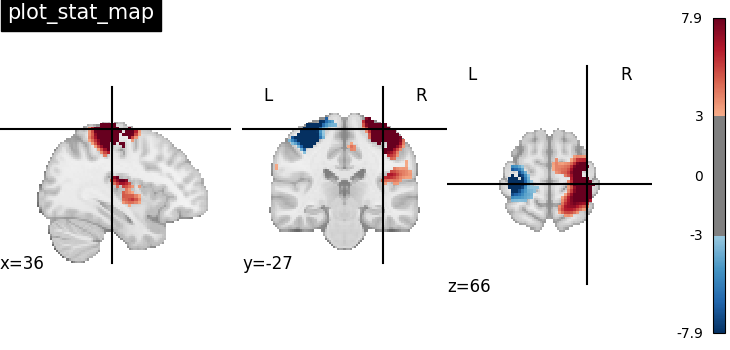Out:

```<nilearn.plotting.displays.OrthoSlicer object at 0x7fc067928730>
```

## 9.2.12.3. Making interactive visualizations with function view_img¶

An alternative to `nilearn.plotting.plot_stat_map` is to use `nilearn.plotting.view_img` that gives more interactive visualizations in a web browser. See Interactive visualization of statistical map slices for more details.

```view = plotting.view_img(stat_img, threshold=3)
# In a Jupyter notebook, if ``view`` is the output of a cell, it will
# be displayed below the cell
view
```

```# uncomment this to open the plot in a web browser:
# view.open_in_browser()
```

## 9.2.12.4. Plotting statistical maps in a glass brain with function plot_glass_brain¶

Now, the t-map image is mapped on glass brain representation where glass brain is always a fixed background template

```plotting.plot_glass_brain(stat_img, title='plot_glass_brain',
threshold=3)
```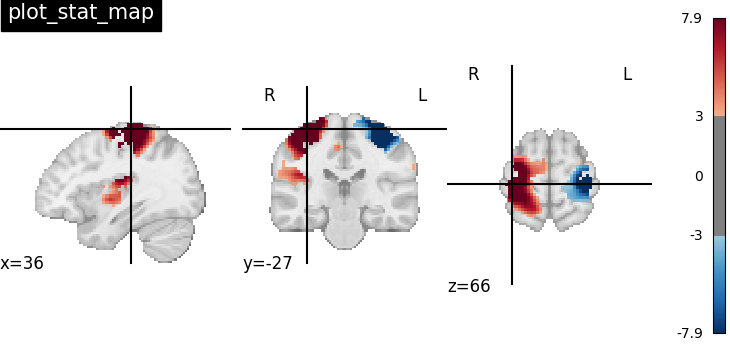Out:

```<nilearn.plotting.displays.OrthoProjector object at 0x7fc02e4cda60>
```

## 9.2.12.5. Plotting anatomical images with function plot_anat¶

Visualizing anatomical image of haxby dataset

```plotting.plot_anat(haxby_anat_filename, title="plot_anat")
```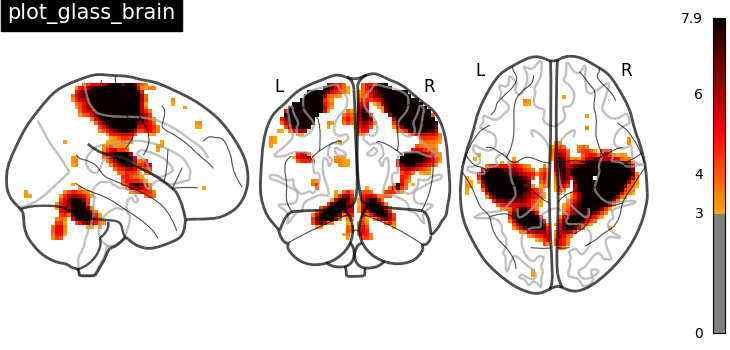Out:

```<nilearn.plotting.displays.OrthoSlicer object at 0x7fc02b9e1370>
```

## 9.2.12.6. Plotting ROIs (here the mask) with function plot_roi¶

Visualizing ventral temporal region image from haxby dataset overlaid on subject specific anatomical image with coordinates positioned automatically on region of interest (roi)

```plotting.plot_roi(haxby_mask_filename, bg_img=haxby_anat_filename,
title="plot_roi")
```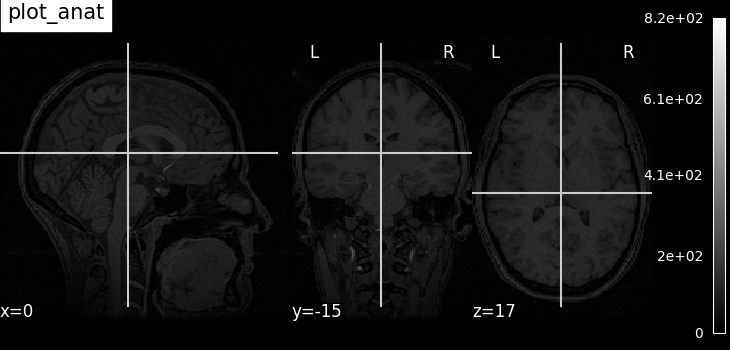Out:

```<nilearn.plotting.displays.OrthoSlicer object at 0x7fc02b744820>
```

## 9.2.12.7. Plotting EPI image with function plot_epi¶

```# Import image processing tool
from nilearn import image

# Compute the voxel_wise mean of functional images across time.
# Basically reducing the functional image from 4D to 3D
mean_haxby_img = image.mean_img(haxby_func_filename)

# Visualizing mean image (3D)
plotting.plot_epi(mean_haxby_img, title="plot_epi")
```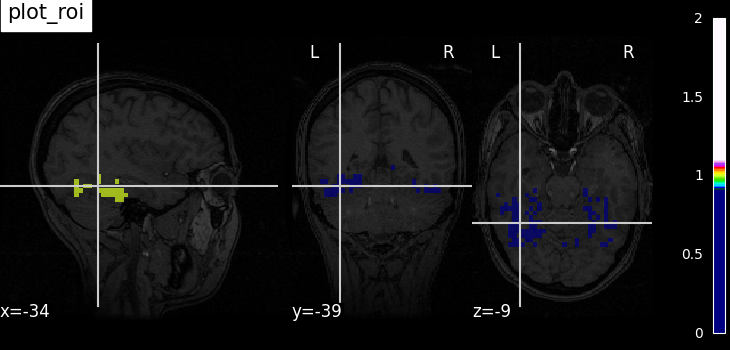Out:

```<nilearn.plotting.displays.OrthoSlicer object at 0x7fc02b8213a0>
```

A call to plotting.show is needed to display the plots when running in script mode (ie outside IPython)

```plotting.show()
```

Total running time of the script: ( 0 minutes 9.082 seconds)

Gallery generated by Sphinx-Gallery